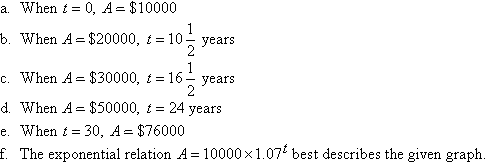Year 10 Interactive Maths - Second Edition

## Exponential Relations

An exponential relation has the form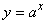where the base number, a, is a positive real number other than 1 and the variable x is the exponent of the base number.

## Exponential Graphs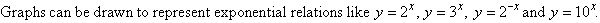#### Example 16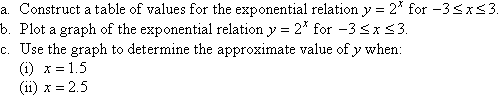##### Solution:

a.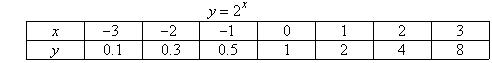b.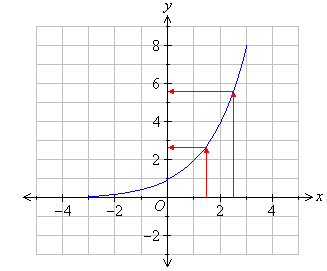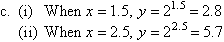Note that the value of y increases rapidly as the value of x increases. This is called exponential growth.

#### Example 17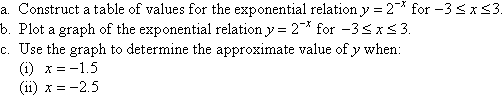##### Solution:

a.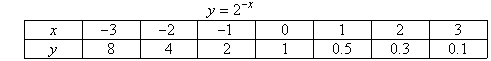b.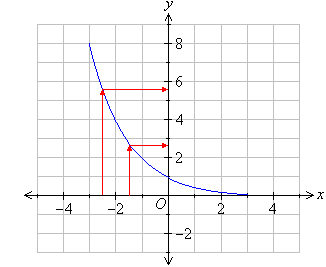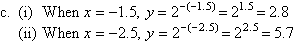Note that the value of y decreases rapidly as the value of x increases. This is called exponential decay.

### Using a Graphics Calculator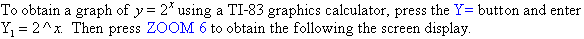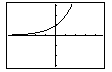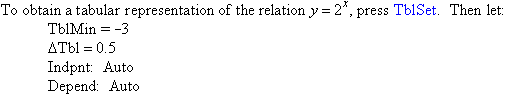Press TABLE to obtain the following screen display.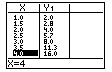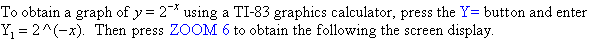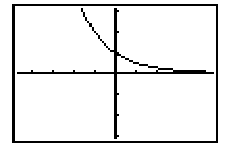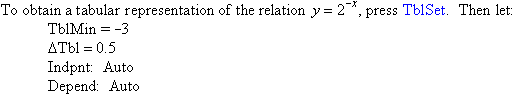Press TABLE to obtain the following screen display.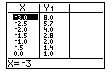### Domain of an Exponential Function

The domain of a function is the set of all first elements (x values) of the function's ordered pairs.

It is clear from the graphs of exponential functions that x can take any value. So, the domain of an exponential function = R (i.e. the set of all real numbers).

### Range of an Exponential Function

The range of a function is the set of all second elements (y values) of the function's ordered pairs.

It is clear from the graphs of exponential functions that y > 0 for all values of x. So, the range of an exponential function = R + (i.e. the set of all positive real numbers).

#### Example 18

The population growth over time (measured in seconds) of a particular bacteria is displayed in the following graph.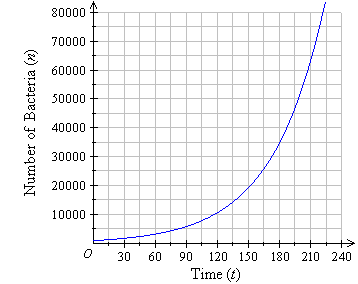a.  How many bacteria are alive after 1 minute?
b.  How many bacteria are alive after 2 minutes?
c.  How many bacteria are alive after 3 minutes?
d.  How many bacteria are alive after 3.5 minutes?
e.  How long did it take the population of bacteria to reach 20000?
f.   How long did it take the population of bacteria to reach 30000?
g.  How long did it take the population of bacteria to reach 35000?
h.  How long did it take the population of bacteria to reach 70000?

##### Solution: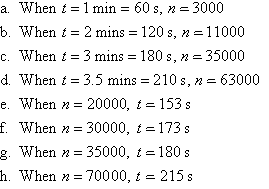#### Example 19

The graph below shows how an investment has grown exponentially over a 30-year period.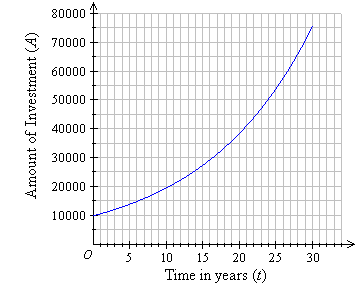a.  What is the initial investment?
b.  How long did it take for the investment value to grow to \$20000?
c.  How long did it take for the investment value to grow to \$30000?
d.  How long did it take for the investment value to grow to \$50000?
e.  What is the value of the investment after 30 years?
f.   Which of the following exponential relations best describes the graph?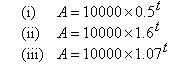##### Solution: